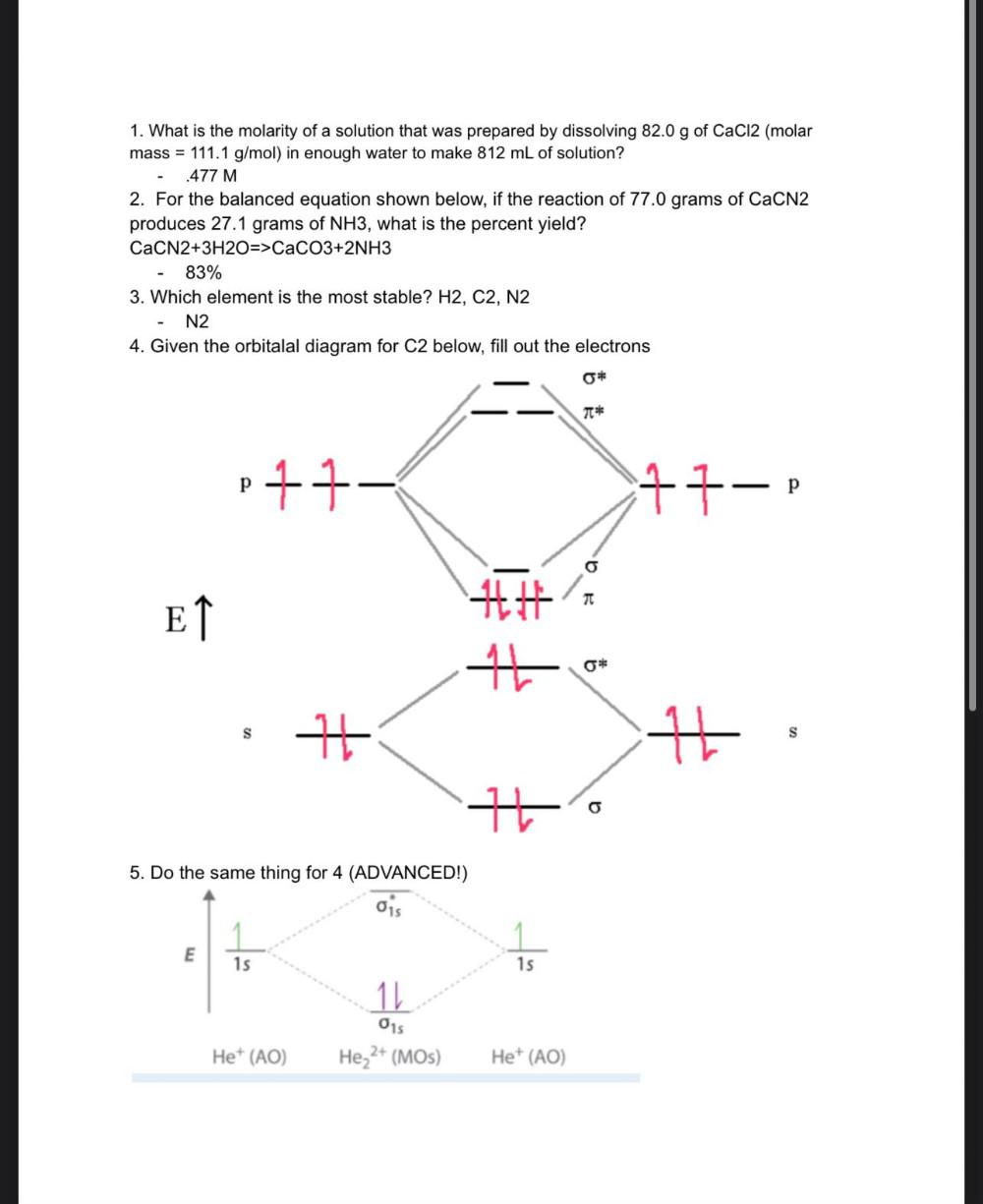Question:

# 1. What is the molarity of a solution that was prepared by dissolving 82.0 g of CaCl2 (molar mass = 111.1 g/mol) in enough water1. What is the molarity of a solution that was prepared by dissolving 82.0 g of CaCl2 (molar mass = 111.1 g/mol) in enough water to make 812 mL of solution? 477 M 2. For the balanced equation shown below, if the reaction of 77.0 grams of CaCN2 produces 27.1 grams of NH3, what is the percent yield? CaCN2+3H2O=>CaCO3+2NH3 83% 3. Which element is the most stable? H2, C2, N2 N2 4. Given the orbitalal diagram for C2 below, fill out the electrons * 7* p 11- 17-p P TT ET 14 16 0* S + 14 s it 5. Do the same thing for 4 (ADVANCED!) 1 E 1s 1s 11 015 Het (AO) He 2+ (MOs) Het (AO)